# 1.2.2 Angles and Lines II, PT3 Practice

Question 6:
In Diagram below, PQRST is a straight line. Find the value of x.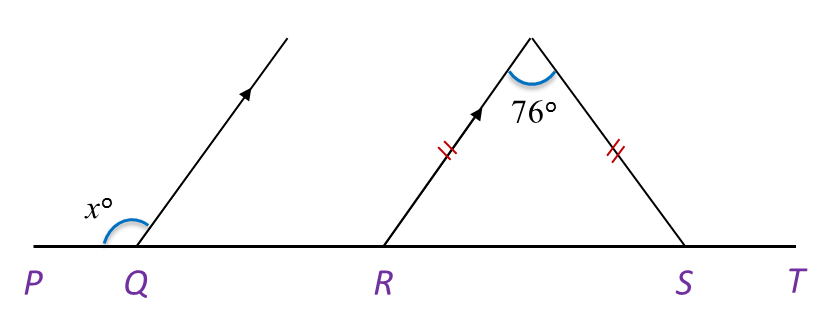Solution:

Question 7:
In Diagram below, find the value of y.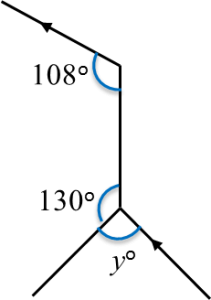Solution:Question 8:
In Diagram below, PSR and QST are straight lines.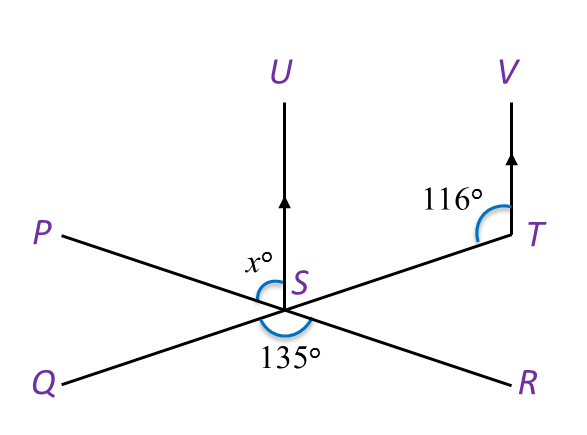Find the value of x.

Solution:
$\begin{array}{l}\angle UST+\angle STV={180}^{o}\\ \angle UST={180}^{o}-{116}^{o}={64}^{o}\\ \angle PST=\angle QSR\\ {x}^{o}+\angle UST={135}^{o}\\ {x}^{o}+\angle UST={135}^{o}\\ {x}^{o}={71}^{o}\\ x=71\end{array}$

Question 9:
In Diagram below, PWV is a straight line.(a) Which line is perpendicular to line PWV?
(b) State the value of  ∠ RWU.

Solution:
(a) SW

(b) ∠ RWU = 13o + 29o + 20o = 62o

Question 10:
In Diagram below, UVW is a straight line.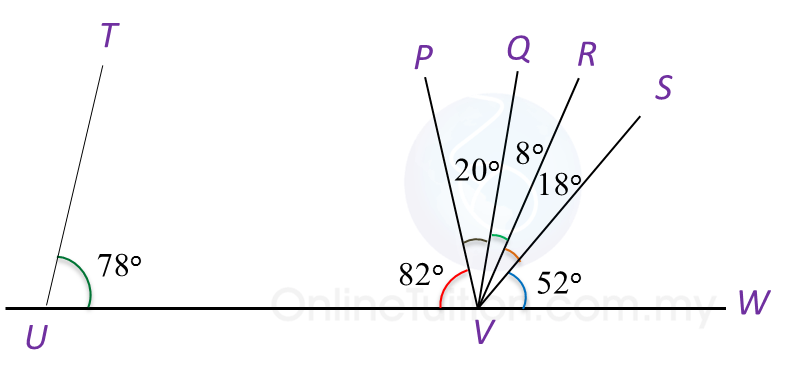(a) Which line is parallel to line TU?
(b) State the value of  ∠ QVS.

Solution:
(a) QV

(b) ∠ QVS = 8o + 18o = 26o

# 12.2.2 Solid Geometry (II), PT3 Focus Practice

                         
12.2.2 Solid Geometry (II), PT3 Focus Practice
 
  Question 5:
  Sphere below has a surface area of 221.76 cm2.Calculate its radius.
                          $\left(\pi =\frac{22}{7}\right)$                        
 
  Solution:
  Surface area of sphere = 4πr2
                          $\begin{array}{l}4\pi {r}^{2}=221.76\\ 4×\frac{22}{7}×{r}^{2}=221.76\\ {r}^{2}=\frac{221.76×7}{4×22}\\ {r}^{2}=17.64\\ r=\sqrt{17.64}\\ r=4.2\text{cm}\end{array}$                      
 
 
 
                         
Question 6:
  Diagram below shows a right pyramid with a square base.Given the height of the pyramid is 4 cm.
  Calculate the total surface area, in cm2, of the right pyramid.
 
  Solution: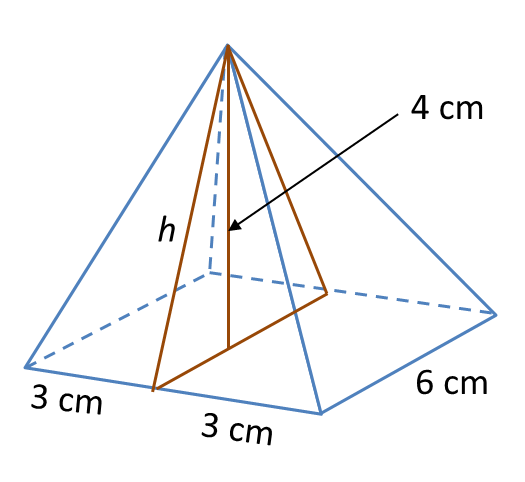h2 = 32 + 42
  = 9 + 16
  = 25
  h = √25   = 5 cm2
 
  Total surface area of the right pyramid
  = Base area + 4 (Area of triangle)
  = (6 × 6) + 4 × 4 (½ × 6 × 5)
  = 36 + 60
  = 96 cm2
 
 
 
         
Question 7:
 
Diagram below shows a prism.Draw to full scale, the net of the prism on the grid in the answer space. The grid has equal squares with sides of 1 unit.
 
 Solution:# 8.2.2 Coordinates, PT3 Focus Practice

Question 6:
The point M (x, 4), is the midpoint of the line joining straight line Q (-2, -3) and R (14, y).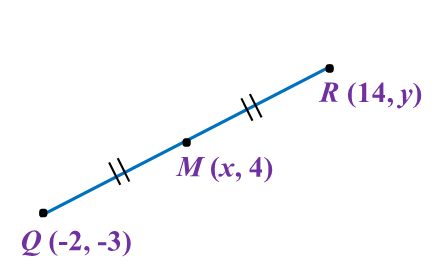The value of x and y are

Solution:

$\begin{array}{l}x=\frac{-2+14}{2}\\ x=\frac{12}{2}\\ x=6\\ \\ 4=\frac{-3+y}{2}\\ 8=-3+y\\ y=11\end{array}$

Question 7:
In diagram below, PQR is a right-angled triangle. The sides QR and PQ are parallel to the y-axis and the x-axis respectively. The length of QR = 6 units.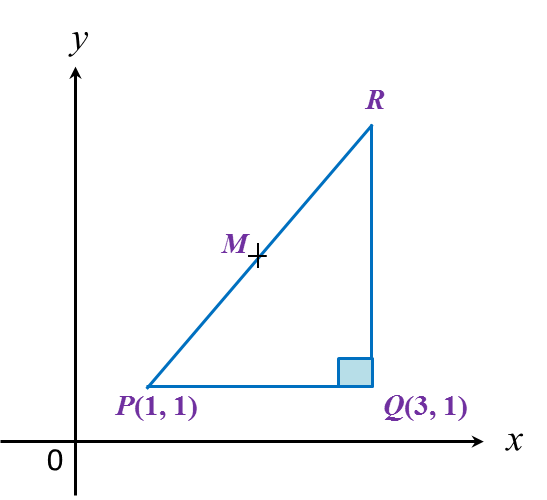Given that M is the midpoint of PR, then the coordinates of M are

Solution:
x-coordinate of R = 3
y-coordinate of R = 1 + 6 = 7
R = (3, 7)

Question 8:
Given points (–2, 8) and (10, 8), find the length of PQ.

Solution:

Question 9:
In diagram below, ABC is an isosceles triangle.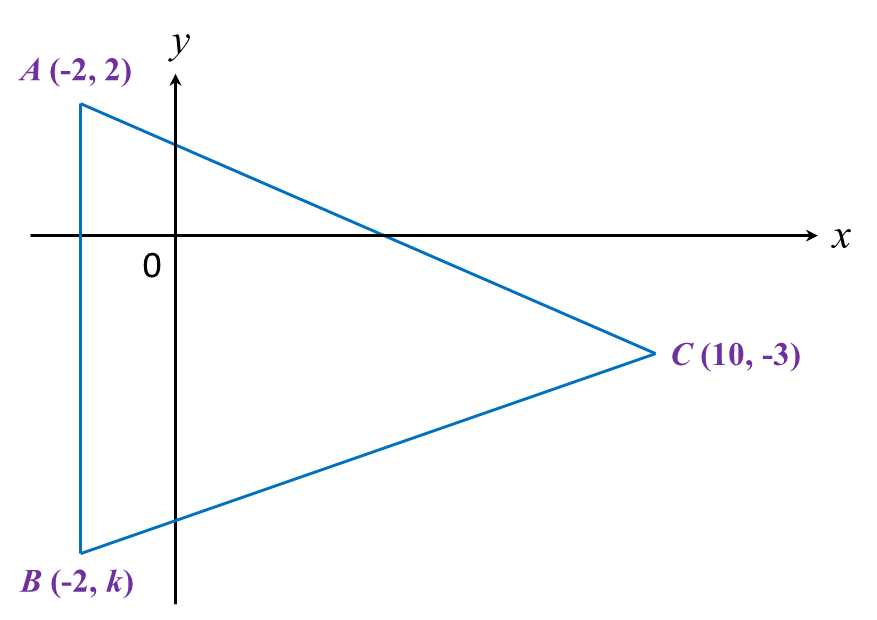Find
(a) the value of k,
(b) the length of BC.

Solution:

Question 10:
Diagram below shows a rhombus PQRS drawn on a Cartesian plane. PS is parallel to x-axis.Given the perimeter of PQRS is 40 units, find the coordinates of point R.

Solution:

# 4.2.2 Linear Equations I, PT3 Practice

Question 6:
Solve each of the following equations:

Solution:
(a)
$\begin{array}{l}5a-16=a\\ 5a-a=16\\ 4a=16\\ a=4\end{array}$

(b)
$\begin{array}{l}6+\frac{2}{3}\left(-9p+12\right)=p\\ 6-6p+8=p\\ -6p-p=-8-6\\ -7p=-14\\ p=2\end{array}$

Question 7::
Solve each of the following equations:

Solution:
(a)
$\begin{array}{l}a-2=\frac{a}{5}\\ 5a-2=a\\ 4a=2\\ a=2\end{array}$

(b)
$\begin{array}{l}\frac{b-3}{4}=\frac{2+b}{5}\\ 5b-15=8+4b\\ 5b-4b=8+15\\ b=23\end{array}$

</div
Question 8:
Solve each of the following equations:

Solution:
(a)
$\begin{array}{l}x=-24-x\\ 2x=-24\\ x=-12\end{array}$

(b)

Question 9:
Solve each of the following equations:

Solution:
(a)
$\begin{array}{l}5p=8p-9\\ 5p-8p=-9\\ -3p=-9\\ p=3\end{array}$

(b)
$\begin{array}{l}3q=\frac{20-13q}{4}\\ 12q=20-13q\\ 12q+13q=20\\ 25q=20\\ q=\frac{4}{5}\end{array}$

# 2.2.2 Number Patterns and Sequences, PT3 Practice

               
Question 6:
 How many prime numbers are there between 10 and 40?
 

 Solution:
 
Prime numbers between 10 and 40
 
= 11, 13, 17, 19, 23, 29, 31, 37
There are 8 prime numbers between 10 and 40.
 
 
 
               
Question 7:
 
Diagram below shows a sequence of prime numbers.
               Find the value of x + y.
 
 Solution:
 
Value of x + y
 
= 19 + 23
 = 42

 
               
Question 8:
 
The following data shows a sequence of prime numbers in ascending order.
               Find the value of x, y and of z.
 
 Solution:
 
x = 11, y = 31, z = 37
 
 
 
       
Question 9:
 
The following data shows a sequence of prime numbers in ascending order.
           Find the value of p, q and of r.
 
 Solution:
 
p = 59, q = 71, r = 79
 
 

 
 
       
Question 10:
 
Diagram below shows several number cards.
            List three prime numbers from the diagram.
 
 Solution:
 
Prime number card from the diagram = 5, 29 and 97.
 
 

 
 

# 2.2.1 Number Patterns and Sequences, PT3 Practice

Question 1:
Diagram below is part of a number line.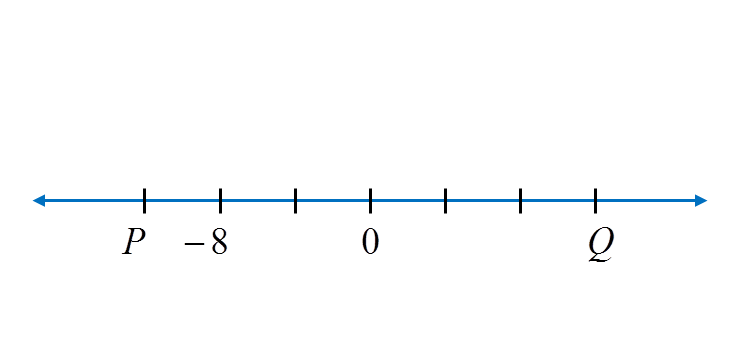What is the value of P and of Q?

Solution:
Each gradation on the number line represents 4 units. Thus P = –12 and Q = 12.

Question 2:
Diagram below shows a sequence of numbers. K and M represent two numbers.
What are the values of K and M?

Solution:K
= 13 – 6 = 7
M = 1 – 6 = –5

Question 3:
Diagram below shows five integers.Find the sum of the largest integer and the smallest integer.

Solution:
The largest integer = 1
The smallest integer = –10
Sum of the largest integer and the smallest integer
= 1 + (–10)
= –9

# 1.2.3 Whole Numbers, PT3 Practice

      
Question 11:
 Britney rents a stall to sell cakes. The rental of the stall is RM500 per month. The cost for baking a cake is RM5 per box and she sells it at RM10 per box. What is the minimum box of cakes must Britney sell each month to make a profit?
 
 Solution:
 
Rental of the stall = RM500 monthly
 Cost of baking a cake per box = RM5
 Selling price per box = RM10
 Profit per box
 = RM10 – RM5
 = RM5
 
 The number of boxes of cakes to accommodate the cost
 = RM500 ÷ RM5
 = 100
 
 Hence, the minimum box of cakes must Britney sell each month to make a profit = 101
 
 
 
 

# 1.2.2 Whole Numbers, PT3 Practice

Question 6:
Solve 4 (8 + 14)(37 – 18) + 46

Solution:
4 (8 + 14)(37 – 18) + 46
= 4(22)(19) + 46
= 4 × 22 × 19 + 46
= 1672 + 46
= 1718

Question 7:
Calculate the value of
113 + 6(47 – 4 × 9)

Solution:
113 + 6(47 – 4 × 9)
= 113 + 6[47 – (4 × 9)]
= 113 + 6(47 – 36)
= 113 + 6(11)
= 113 + 66
= 179

Question 8:
Solve 128 + (9 × 4) – 318 ÷ 6

Solution:
128 + (9 × 4) – 318 ÷ 6
= 128 + 36 – 53
= 164 – 53
= 111

Question 9:
Calculate the value of
62 × 15 – 40 + 68 ÷ 17.

Solution:
(62 × 15) – 40 + (68 ÷ 17)
= 930 – 40 + 4
= 894

Question 10:
402 – ( ) = 78 – 6 × 8 ÷ 16.
Find the missing number in the box.

Solution:
402 – ( ) = 78 – 6 × 8 ÷ 16
402 – ( ) = 78 – 48 ÷ 16
402 – ( ) = 78 – 3
402 – ( ) = 75
( ) = 402 – 75
( ) = 327

# 1.2.1 Whole Numbers, PT3 Practice

     
Question 1:
 Solve 8 × 2 ÷ 4 + 12.
 

 Solution:
 
8 × 2 ÷ 4 + 12
 = 16 ÷ 4 + 12
 = 4 + 12
 = 16
 
 
 
      
Question 2:
 Solve 7(6 + 5) – 3(13 – 9).
 

 Solution:
 
7(6 + 5) – 3(13 – 9)
 = 7(11) – 3(4)
 = 77 – 12
 = 65
 
 
 
     
Question 3:
 Solve 64 + (136 – 87) × (28 ÷ 4).
 

 Solution:
 
64 + (136 – 87) × (28 ÷ 4)
 = 64 + 49 × 7
 = 64 + 343
 = 407
 
 
 
    
Question 4:
 Solve 84 ÷ 4 – 9 + 6 × 7
 

 Solution:
 
84 ÷ 4 – 9 + 6 × 7
 = 21 – 9 + 42
 = 12 + 42
 = 54
 
 
 
    
Question 5:
 Solve 92 + 13 × 5 – 42
 

 Solution:
 
92 + 13 × 5 – 42
 = 92 + (13 × 5) – 42
 = 92 + 65 – 42
 = 157 – 42
 = 115
 
 
 
 

# Element and Compound

Matter can be divided into elements and  compounds.### Elements

1. An element is a substance that consists of only one type of atom.
2. Element can be either atoms or molecules.
Example:
(Both the iron and oxygen are element because they consist of only one type of atoms)
##### Compounds
1. A compound is a substance composed of molecules made up of atoms of two or more elements.
2. A compound is made up of either molecules or ions.
Example:
(Both the sodium chloride and carbon dioxide are compound because they consist of more than one type of atoms)